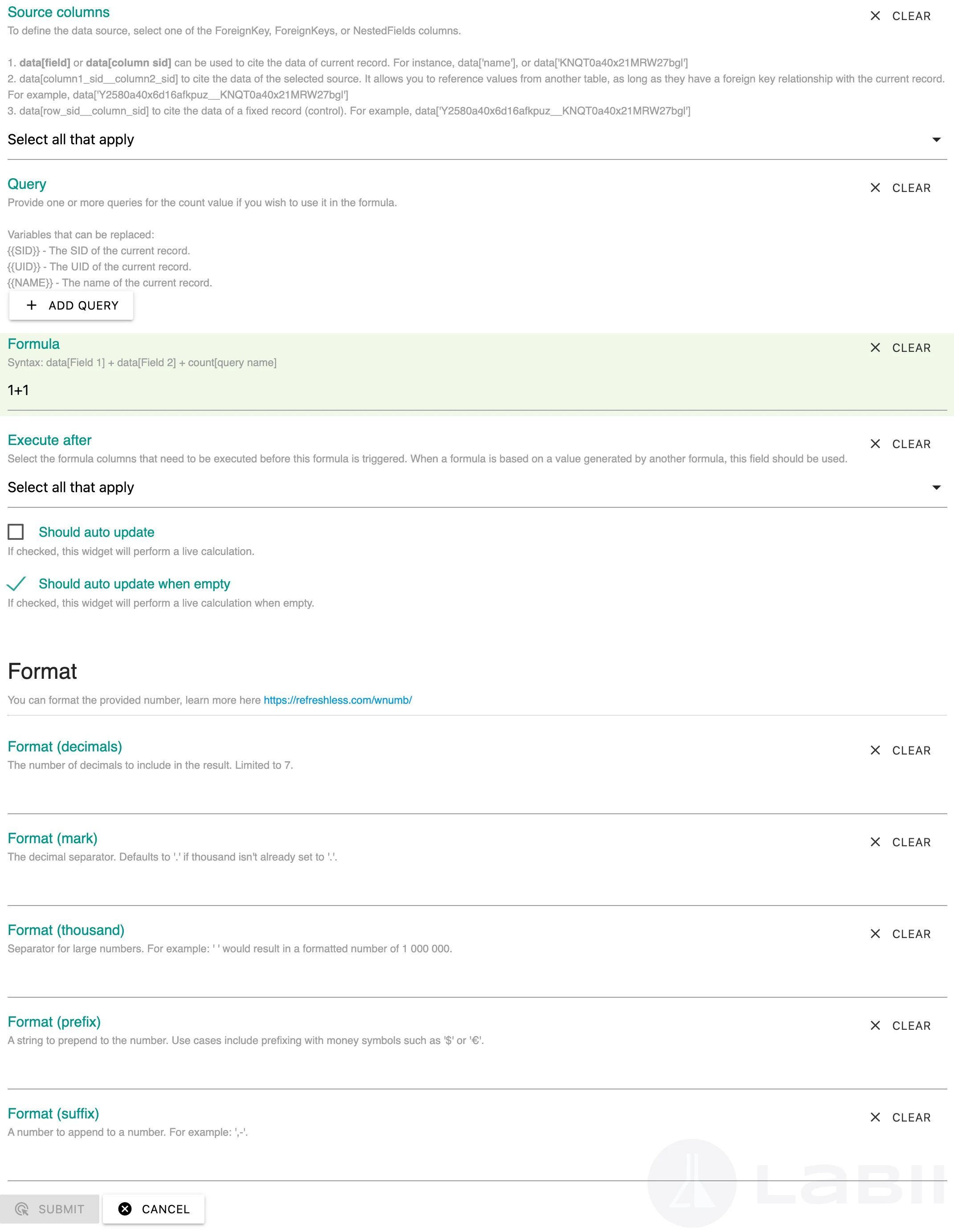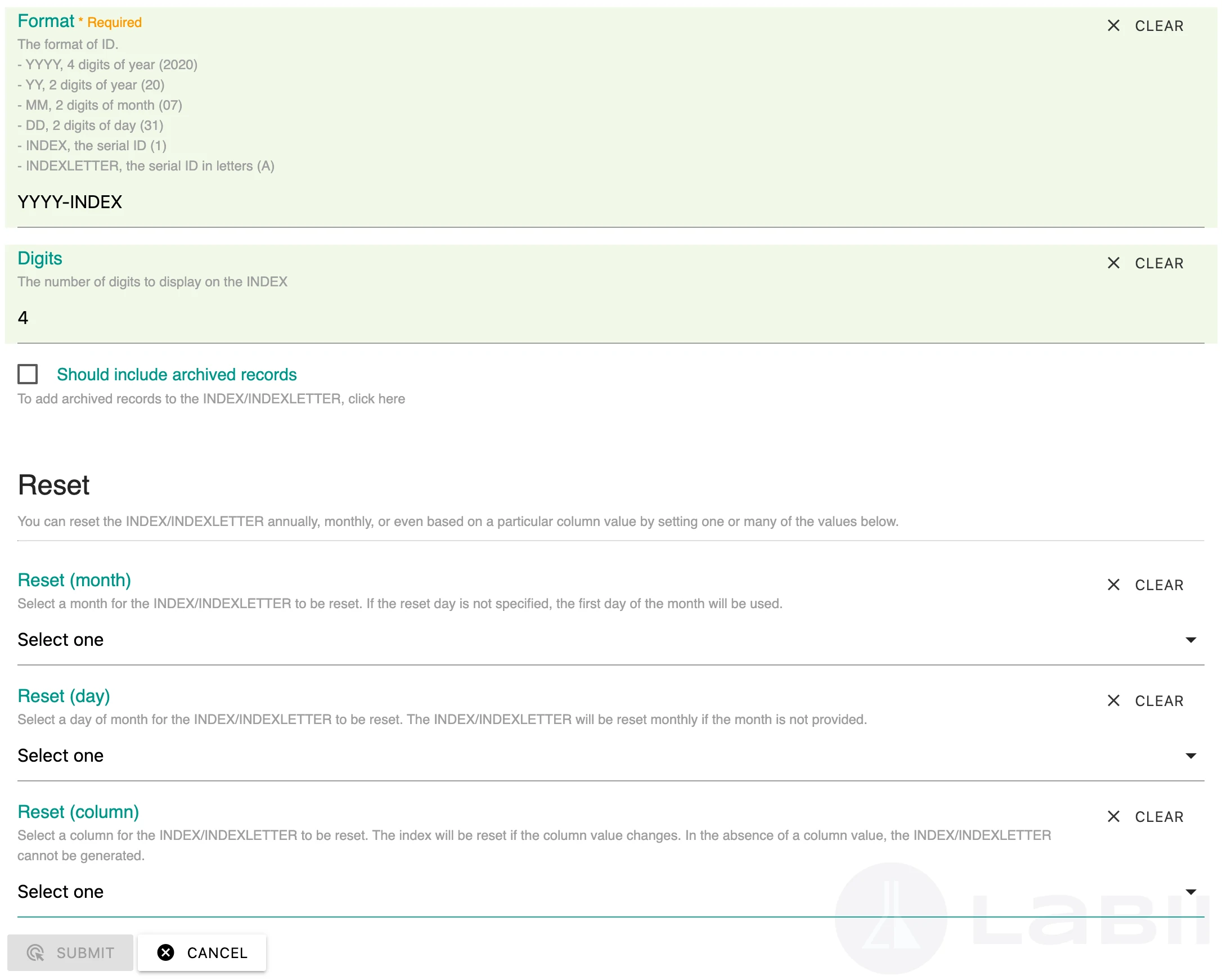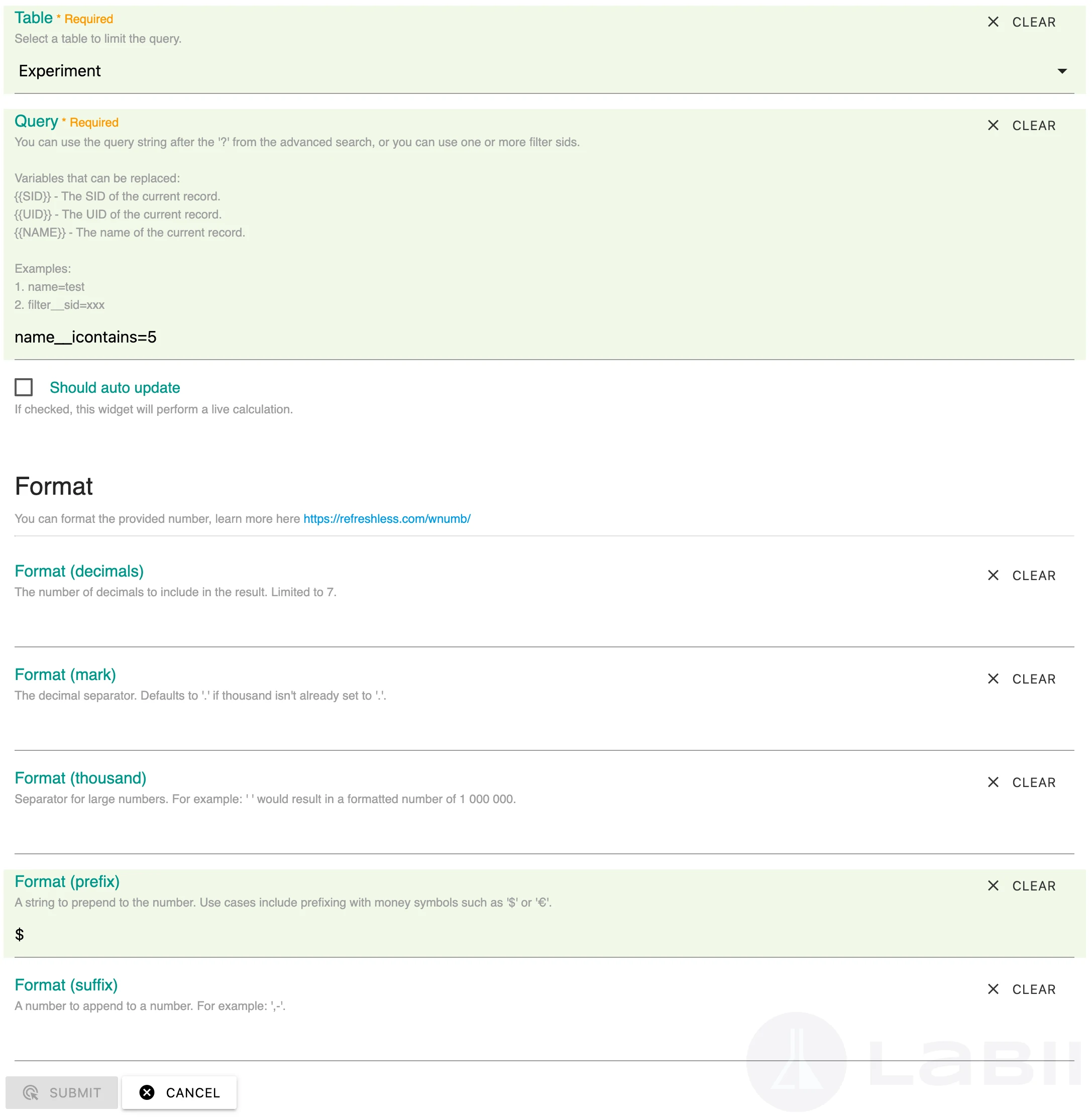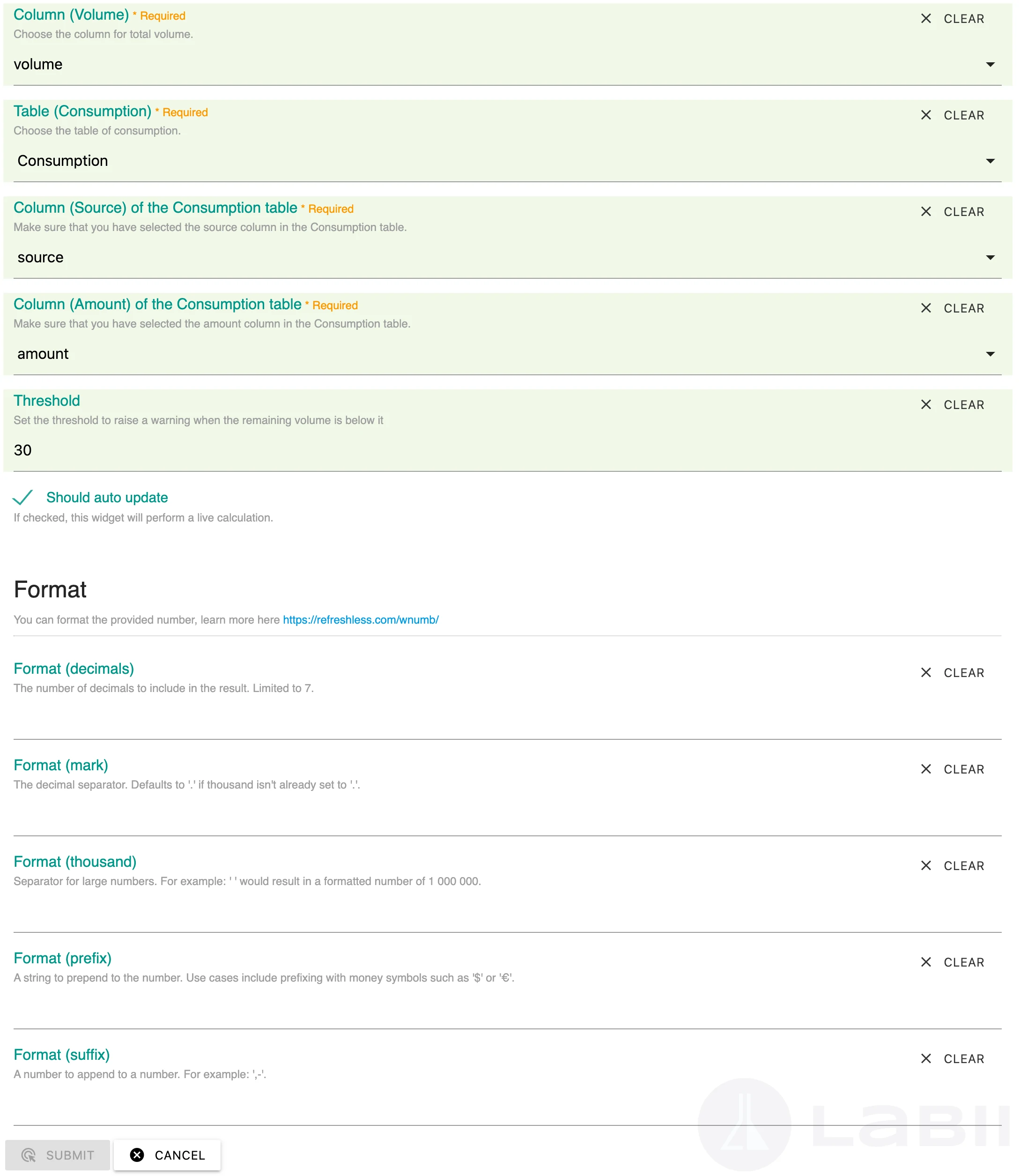# Calculation

Calculation related column widgets.

## Overview

You can perform related calculations using the widget in this category.

## Formula

Use this widget to display value calculated based on a formula. The formula shall be defined in the Default Value of the column.Edit view:
Not supported
Form view:
Not supported

#### Configuration{
"columns": [ # (optional),
{"sid":"xxx", "name":"xxx"}, # The column sid of ForeignKey, ForeignKeys, NestedFields, optional
],
"queries": [ # (optional)
{
"name": "xxx",
"table": {"sid":"xxx", "name":"xxx"},
"query": "name=xxx"
}
],
"formula": "", # (required), the formula, see the Syntax below
# data[column_sid], value of a column of current row
# data[row_sid__column_sid], value of column of a fixed row
# data[`\${data['column__sid'].sid}`__column_sid]
# data[column1_sid__column2_sid], column1_sid, the ForeignKey column listed in rows;
# data[column1_sid__column2_sid] of ForeignKeys returns array of data.
# count[table_sid__column_sid], the count value
"after": [], # Select the formula columns that need to be executed before this formula is triggered.
# When a formula is based on a value generated by another formula, this field should be used.
"format": {}, # (optional), the number in the wNumb format.
"should_auto_update": false, # (optional), default to false.
# If true, the widget will perform live calculation
# If false, the user have to click reload to update the value
"should_auto_update_when_empty": true, # (optional), default to true.
# If true, the widget will perform live calculation when the data is empty
# If false, the results will not be calculated on default
}

#### Variables

• data[column_sid], value of a column of current row
• data[row_sid__column_sid], value of column of a fixed row
• data[`\${data['column__sid'].sid}`__column_sid]
• data[column1_sid__column2_sid], column1_sid, the ForeignKey column listed in rows;
• data[column1_sid__column2_sid] of ForeignKeys returns array of data.
• count[table_sid__column_sid], the count value

#### Syntax

`data[Field 1] + data[Field 2] + data[Source column sid__Target solumn sid]`
Wheres, Field is the SID of a column. For example:
• `data[8adg0a40x52520ejotyDI] + data[69be0a40x52508chmrwBG]`
• `data[8adg0a40x52520ejotyDI].substring(1,2)`
• `data[8adg0a40x52520ejotyDI].substring(1,3) == "ATCG" ? "Good" : "Bad"`
• `data[8adg0a40x52520ejotyDI__69be0a40x52508chmrwBG].substring(1,2)`
Labii also supports multiple functions, it must include a `return` line:
var a = 1;
var b = 2;
return a + b;

#### Operator

• + Addition, `a + b`
• - Subtraction,
• * Multiplication
• ** Exponentiation (ES2016)
• / Division
• % Modulus (Remainder)
• sum - get sum value of array
• mean - get mean value of array
• sd - get standard deviation of array
• se - get standard error of array
• Priority calculate with `()` is supported, `(100 + 50) * a;`
• If condition: `true ? a : b`, if the condition is true, then return a, else, return b
• Substring: `string.substring(start, end)`
• Case:
• Upper: str.toUpperCase()
• Lower: str.toLowerCase()
• Date: `new Date(dateString)`, learn more at here.
• Math:
• abs(x) Returns the absolute value of x
• acos(x) Returns the arccosine of x, in radians
• asin(x) Returns the arcsine of x, in radians
• atan(x) Returns the arctangent of x as a numeric value between -PI/2 and PI/2 radians
• atan2(y, x) Returns the arctangent of the quotient of its arguments
• ceil(x) Returns the value of x rounded up to its nearest integer
• cos(x) Returns the cosine of x (x is in radians)
• exp(x) Returns the value of Ex
• floor(x) Returns the value of x rounded down to its nearest integer
• log(x) Returns the natural logarithm (base E) of x
• max(x, y, z, ..., n) Returns the number with the highest value
• min(x, y, z, ..., n) Returns the number with the lowest value
• pow(x, y) Returns the value of x to the power of y
• random() Returns a random number between 0 and 1
• round(x) Returns the value of x rounded to its nearest integer
• sin(x) Returns the sine of x (x is in radians)
• sqrt(x) Returns the square root of x
• tan(x) Returns the tangent of an angle
Import value:
Not supported

## Numbering

Use this widget to generate your own ID with auto-increment. The Numbering can be generated automatically and can also be manually updated.Click the reload button to update the ID
Edit view:
Not supported
Form view:
Not supported
Configuration{
"format": "YY-ID", # (required), the format of the id
# YYYY - 2020, 4 digits of year
# YY - 20, 2 digits of year
# MM - 07, 2 digits of month
# DD - 01, 2 digits of day
# ID, the serial id
# FOREIGNKEYCOLUMN - refer to the data from a foreignkey
"digits": 4, # (optional), number of digits of ID to display
"should_include_archived_records": false, # (optional), whether to add archived records to the INDEX/INDEXLETTER
"reset_month": 10, # (optional), the month to reset the id
"reset_day": 1, # (optional), the day to reset the id
"reset_column": {"sid":"xxx", "name":"xxx"}, # (optional), the column to reset the ID
}
The default value will be ignored for the ID widget.
Import value:
The ID value.

## RowCount

Use this widget to display the count of a Row. For example, the number of biospecimens generated from a host.
To make it work, provide the query string into column configuration.Edit view:
Not supported
Form view:
Not supported
Configuration{
"table": {"sid":"xxx", "name":"xxx"}, # the table to limit the query
"query": "name__icontains=test", # (requried)
# paste the query
# Some variables can be added to the link. The widget will replace it for you:
# {{SID}}, the sid of the current record.
# {{UID}}, the uid of the current record.
# {{NAME}}, the name of the current record.
"should_auto_update": false, # default to false.
# If true, the widget will perform live calculation
# If false, the user have to click reload to update the value
"format": {}, # the number in the wNumb format.
}
Import value:
Not supported

## Consumption

Use this widget to display the remaining volume of a sample or reagent.Edit view:
Not supported
Form view:
Not supported
Configuration:• Column "Volume Value" with Number widget
• Table "Consumption"
• Column "Sample" or "Reagent" with ForeignKey widget
• Column "Amount" with Number widget
{
"volume": {"sid":"xxx", "name":"xxx"},
# required, the sid of the column "Volume Value"
"consumption": {"sid":"xxx", "name":"xxx"},
"source": {"sid":"xxx", "name":"xxx"},
# required, the sid of the column "Sample" or "Reagent" from the consumption table
"amount": {"sid":"xxx", "name":"xxx"},
# required, the sid of the column "Amount" from the consumption table
"threshold": 10,
# the color of the value will turn red if the remaining value lower than the threshold
"should_auto_update": true, # default to true.
# If true, the widget will perform live calculation
# If false, the user have to click reload to update the value
"format": {}, # the number in the wNumb format.